## Apache Spark and Scala

Certification Training
5898 Learners
7 Chapters +

# Apache Spark Graphx Programming Tutorial

Welcome to the last chapter, Chapter 7, of the Apache Spark and Scala tutorial (part of the Apache Spark and Scala course). This Chapter will introduce and explain the concepts of Spark GraphX programming.

Let us explore the objectives of Apache Scala in the next section.

## Objectives

After completing this lesson, you will be able to:

• Explain the fundamental concepts of Spark GraphX programming

• Discuss the limitations of the Graph Parallel system

• Describe the operations with a graph, and

• Discuss the Graph system optimizations

We will begin with an introduction to Graph-Parallel System in the next section.

## Introduction to Graph-Parallel System

Today, big graphs exist in various important applications, be it web, advertising, or social networks. A few of such graphs are represented graphically below.

These graphs allow performing tasks such as targeting advertising, identifying communities, and deciphering the documents meaning. This is possible by modeling the relations between products, users, and ideas. The size and significance of graph data are growing. In its response, various new large-scale distributed graph-parallel frameworks, such as GraphLab, Giraph, and PowerGraph, have been developed.

With each framework, a new programming abstraction is available. These abstractions allow to explain graph algorithms in a compact manner and also, the related runtime engine that can execute these algorithms efficiently on distributed and multicore systems.

Additionally, these frameworks abstract away the issues of the large-scale distributed system design. Therefore, they are capable of simplifying the design, application, and implementation of the new sophisticated graph algorithms to large-scale real-world graph problems.

In the next section of apache spark and scala tutorial, we will discuss limitations of Graph-Parallel System.

Interested in learning more about Apache Spark & Scala? Enroll in our Apache course today!

## Limitations of Graph-Parallel System

Before we move further, you should know the limitations of the Graph-Parallel system.

One of them is that although the current frameworks have various common properties, each of them presents a little different graph computation. These computations are custom-made for a specific graph applications and algorithms family or the original domain.

Additionally, all these frameworks depend on a different runtime. Therefore, it is tricky to create these abstractions.

While these frameworks are capable of resolving the graph computation issues, they cannot resolve the data ETL issues. They also cannot address the issues related to the process of deciphering and applying the computation results. The new frameworks however have built-in support available for interactive graph computation.

In the next section of the tutorial, we will begin with an introduction to GraphX.

## Introduction to GraphX

Let’s now talk about GraphX, which is a graph computation system running in the framework of the data-parallel system. It extends the RDD abstraction and hence introduces a new feature called Resilient Distributed Graph or RDG. In a graph, RDG relates records with vertices and edges and produces an expressive computational primitives’ collection.

In addition, it simplifies the graph ETL and analysis process substantially by providing new operations for viewing, filtering, and transforming graphs.

GraphX combines the benefits of graph-parallel and data-parallel systems as it efficiently expresses graph computation within the framework of the data-parallel system. In addition, GraphX distributes graphs efficiently as tabular data structures by leveraging new ideas in their representations. In a similar way,

GraphX uses in-memory computation and fault-tolerance by leveraging the improvements of the data flow systems. GraphX also simplifies the graph construction and transformation process by providing powerful new operations. With the use of these primitives, it is possible to implement the abstractions of PowerGraph and Pregal in a few lines. It is also possible to load, transform, and compute interactively on massive graphs.

The image below shows how GraphX works.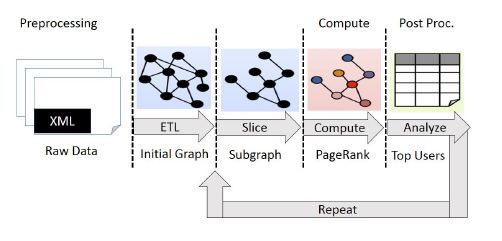In the next section of the tutorial, we will discuss importing GraphX.

## Importing GraphX

To start working with GraphX, you first need to import it and Spark into your project. The code to do this is given below.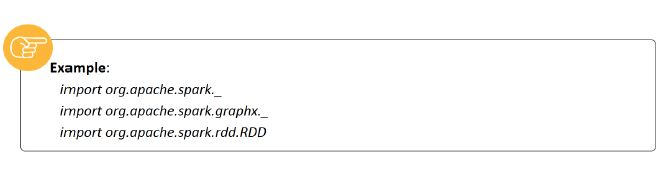We will discuss the property graph and its features in the next subsequent section of the tutorial.

## The Property Graph

The property graph is defined as a directed multigraph that has properties related to every vertex and edge. Here, a directed graph is defined as a graph that has potentially various parallel edges that share the same source and destination vertexes.

Every vertex is identified by a unique 64-bit long identifier, known as VertexID. In a similar manner, every edge has an individual source and destination vertex identifier. The properties of these graphs are saved as Scala or Java objects along with their every vertex and edge.

These graphs are parameterized over the edge or ED and vertex or VD types. Here, the types are the types of objects that are related to every edge and vertex. GraphX reduces the memory footprint by optimizing the presentation of edge and vertex types when they exist as plain old data types and by saving them in specialized arrays.

The code given below shows the same.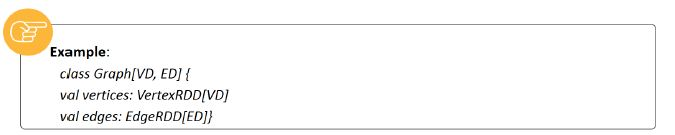Here, this class extends and is an optimized version of RDD[(VertexID, VD)]; however, this class is an optimized version of RDD[Edge[ED]]. Both VertexRDD[VD] and EdgeRDD[ED] leverage internal optimizations and offer additional functionality that is built around graph computation.

An example of the property graph is displayed below.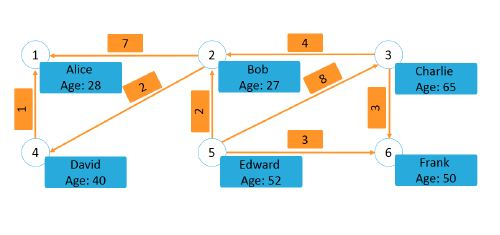## Features of the Property Graph

A few more features of the property graph are also listed on the screen.

Similar to RDDs, the property graph is also fault-tolerant, distributed, and immutable. If you need to perform any changes to the structure or values of the graph, you would need to produce a new graph with the required changes. Note that there are considerable parts of the original graph, which include structure, indices, and attributes, which remain unaffected. These parts are reused in the new graph, which reduces this inherently functional data-structure cost.

You can use various vertex-partitioning heuristics to partition the graph across the workers. Similar to RDDs, every graph partition can be created again on a separate machine in case a failure happens.

From the logical standpoint, the property graph is similar to a typed collections RDDs pair that encodes each vertex and edge properties. As a result, it includes members for accessing the graph vertices and edges.

In the next section of the tutorial, we will discuss how to create a graph.

## Creating a Graph

Now, let us understand how to create a graph. The code to create a simple graph of a co-worker is given below. A graphical representation of this graph is also given below.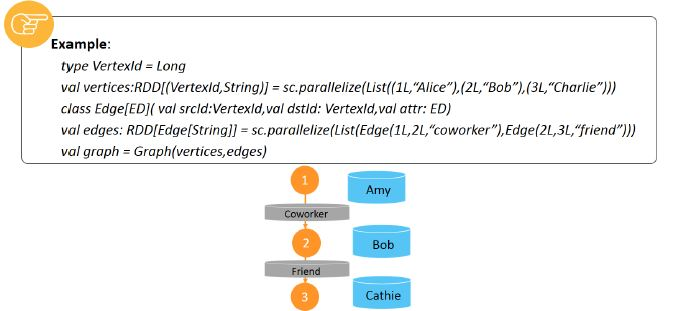In the next section of the tutorial, we will discuss Triplet View.

## Triplet View

Apart from the property graph’s vertex and edge views, GraphX also includes a triplet view. This view combines the properties of the vertices and edges logically that produce the given class. This class contains the EdgeTriplet class instances.

The EdgeTriplet class adds the given members containing the source and destination properties respectively and hence extends the Edge class.

This view is also shown graphically below.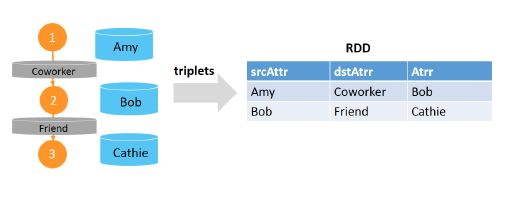In the next section of the tutorial, we will discuss Graph Operators.

## Graph Operators

Similar to RDDs, property graphs also provide various basic operators.

These operators input user-defined functions and result in new graphs that have properties and structures transformed. The core operators with optimized implementations are defined in a graph. On the other hand, the convenient operators expressed as core operators compositions are defined in GraphOps. However, the GraphOps operators are available as Graph members automatically because of Scala implicit.

To understand this, consider the given code example that can compute the in-degree of every vertex that is defined in GraphOps.

The reason why core graph operators are differentiated from GraphOps is to be able for supporting various future graph representations.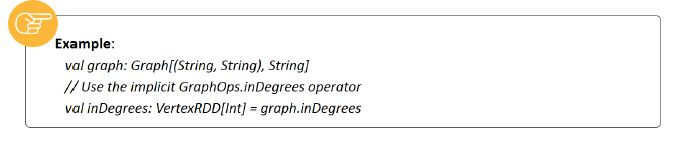We will discuss the list of operators in the next subsequent section of the tutorial.

## List of Operators

The code shown below shows a functionality summary of the operators defined in Graph and GraphOps.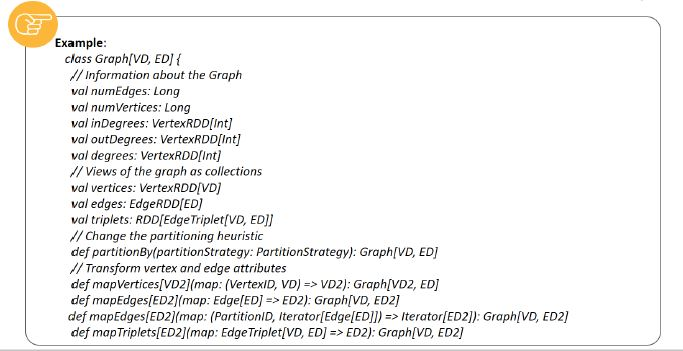For simplicity, these are presented as graph members. You should note that a few function signatures have been simplified and a few more advanced functionalities have been removed. Therefore, you should refer to the API docs to determine the official list of operations.

The further code is displayed.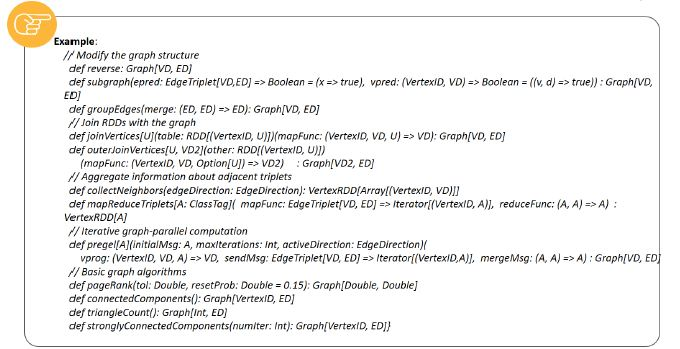## Property Operators

Similar to the map operator of RDDs, the property graph also contains property operators. The code to define and use them is displayed below. These operators are generally used for initializing the graph for a specific project or computation.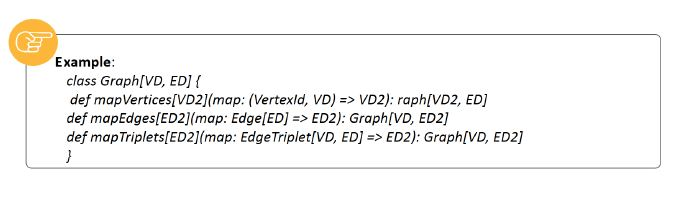## Structural Operators

At present, GraphX provides support to just commonly used structural operators; however, more are expected to be added in the future. The supported ones include reverse operators and subgraph operators. The use of these operators is explained through the given code.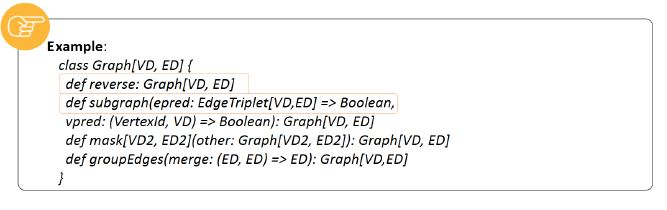The reverse operators reverse all the edge directions and return new graphs. For instance, they can be used in case of computing the inverse PageRank. These operators do not change the properties of vertices and edges and the edges number. Therefore, they can be used without data duplication or movement efficiently.

On the other hand, the subgraph operators input the predicates of vertices and edges and return graphs that contain only the vertices satisfying the vertex predicate and edges satisfying the edge predicate.

They also connect the vertices satisfying the vertex predicate. These operators are used for restricting the graph to the suitable vertices and edges by eliminating the broken links.## Subgraphs

Let’s learn more about subgraphs. In the first image shown below, this operator is being used to return the graph that contains only those vertices where the relation type is not “relative”.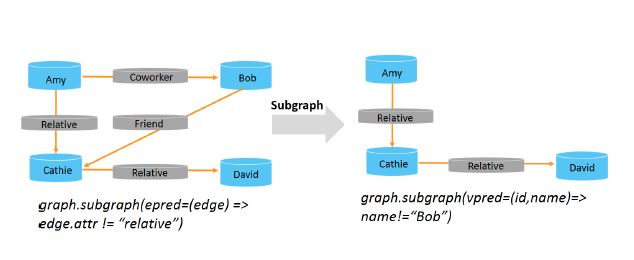However, in the second image, it is being used to return the graph that contains only those vertices who value is Bob.

## Join Operators

Sometimes, it is required to join data originating from RDDs or external collections that have graphs.

For instance, in cases when you need to pull the vertex properties from one graph to the other, you might require extra properties. In such cases, join operators are useful. The supported ones include joinVertices operator and outerJoinVertices operators. The use of these operators is explained through the given code.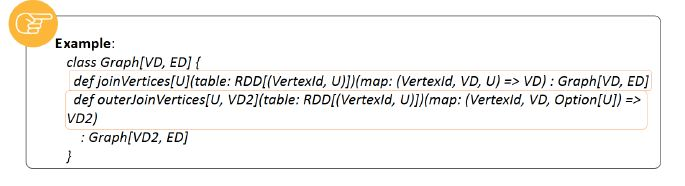The joinVertices operator is capable of joining the vertices with an RDD. It then returns a graph having its vertex properties received by the application of the user-defined map function to the joined vertices result. For the vertices with a matching value in the RDD, the original value is retained.

On the other hand, the outerJoinVertices operator is more general and operates similarly to joinVertices. The only difference is that the user-defined map function is applied to all vertices. It can alter the type of vertex property. The map function takes an Optiontype, as all vertices may not have a matching value in the RDD being inputted.

In the next section of the tutorial, we will discuss neighborhood aggregation.

## Neighborhood Aggregation

An important step in various graph analytics tasks is to aggregate the neighborhood information of every vertex. For instance, you might require identifying the number of every user’s followers. Various iterative graph algorithms such as Shortest Path and PageRank perform this operation.

The primary aggregation operator, mapReduceTriplets, inputs a user-defined map function applied to every triplet and then provides messages that are destined to none, both, or either vertices in the triplet. Its use is as depicted in the given code.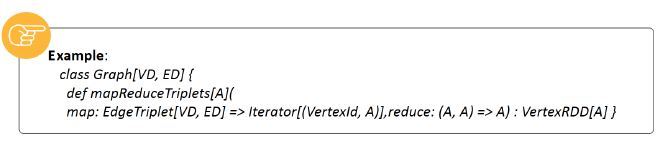For improving performance, this primary operator has been changed to the new graph.AggregateMessages operator.

In the next section of the tutorial, we will discuss mapReduceTriplets.

## MapReduceTriplets

Let’s discuss more primary aggregation operator, mapReduceTriplets.

As discussed, with this operator, the map function is applied to every edge graph triplet. The messages thus yielded are destined to the vertices that are adjacent. With the reduce function, messages that are destined to the same vertex are aggregated. As a result, a VertexRDD is obtained that contains aggregate messages for every vertex.

For instance, consider the given code in which mapReduceTriplets is being used for counting the number of degree for each vertex.

The image below also shows the application of this operator.We will discuss the counting degree of the vertex in the next section of the tutorial.

## Counting Degree of Vertex

One of the common aggregation tasks is to compute the degree of every vertex, which is defined as the number of edges that are adjacent to every vertex. When it comes to directed graphs, it is generally required to identify the out-degree, in-degree, and the total degree of every vertex. The operators to compute these degrees of every vertex are included in the GraphOps class.

For instance, consider the given code that is computing the maximum in, out, and total degrees.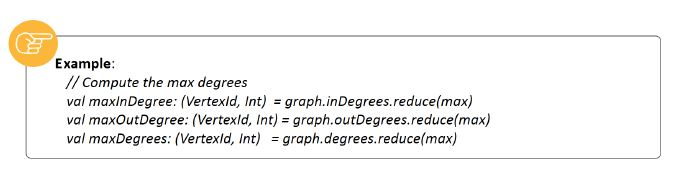In the next section of the tutorial, we will discuss collecting neighbors.

## Collecting Neighbors

Sometimes, it is easy to express computation by performing a collection of neighboring vertices and the related attribute at every vertex. To do so, you can use the given operators. The code to use them is given below.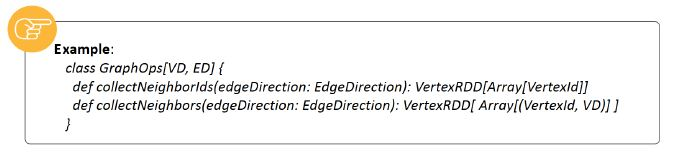These operators can prove to be very costly because they need substantial communication and duplicate information. If possible, try to express the same computation by the use of the aggregateMessages operator.

In the next section of the tutorial, we will discuss Caching and Uncaching.

## Caching and Uncaching

Similar to RDDs, GraphX must be cached explicitly when using multiple times, as they are not persisted in memory by default. Therefore, you should always call the Graph.cache() method first.

In case of iterative computations, you may also need to uncache to obtain the best performance. Cached graphs and RDDs, by default, exist in memory until a pressure evicts in an LRU order. In such computations, intermediate results originating from previous computations fill the cache.

However, they get evicted eventually, the data that is unnecessarily stored in memory slows down garbage collection. Therefore, it is more efficient if you uncache these intermediate results as soon as they are not required. This includes uncaching all other datasets, materializing graphs or RDDs, and using only the materialized datasets for further iterations.

Graphs include various RDDs and therefore, it is tricky to unpersist them correctly. In case of iterative computations, you should use the Pregel API that unpersists intermediate results correctly.

We will discuss graph builders in the next section of the tutorial.

## Graph Builders

To build a graph from a vertices and edges collection existing on a disk or in an RDD, GraphX provides various ways. By default, none of these graph builders repartitions the edges of a graph. Instead, those are left in their as is default partitions.

These graph builders are listed below.

Graph.groupEdges

Graph.groupEdges needs that the graph should be repartitioned. This is because of its assumption that identical edges are collocated on the same partition. Therefore, before calling this, you must call Graph.partitionBy.

Graph.apply

The next graph builder, Graph.apply, lets you create a graph from RDDs containing vertices and edges. It picks duplicate vertices arbitrarily. It also picks the vertices that are found in the edge RDD, but does not pick the vertex RDD that is assigned the default attribute.

Graph.fromEdges

The Graph.fromEdges builder lets you create a graph only from an RDD of edges. It creates any vertices mentioned by edges automatically and assigns them the default value.

Graph.fromEdgeTuples

With the Graph.fromEdgeTuples graph builder, you can create a graph only from an RDD of edge tuples.

This assigns the value 1 to the edges and then creates any vertices mentioned by edges automatically while assigning them the default value.

This graph builder also provides support to deduplicate the edges. For this, you would need to pass some of a PartitionStrategy as the uniqueEdges parameter. It also requires a partition strategy to similar collocate edges on the same partition in order to deduplicate them.

In the next section of the tutorial, we will discuss vertex and edge RDDs.

## Vertex and Edge RDDs

Another concept related to GraphX is vertex RDDs. The VertexRDD[A] is an extension of the given class.
It adds additional constraints that every VertexID appears just once. In addition, it represents a vertices set, where each vertex has an attribute of type A. This is accomplished by saving the attributes of vertices in a hash-map and reusable data structure. As a result, two VertexRDDs can be combined in constant time with no hash evaluations if they are derived from the same base.

Similarly, the EdgeRDD[ED] is an extension of the given class. It organizes the edges into blocks that are partitioned by the use of one of the partitioning strategies that are defined in PartitionStrategy. The attributes of edges and the adjacency structure are saved differently that enables the maximum reuse when it comes to the changing attribute values. The use of three additional functions exposed by it is explained through the given code.

Generally, the operations on the Edge RDDs are achieved by the use of graph operators, or they depend upon the operations that are defined in the base RDD class.

In the next section of the tutorial, we will discuss Graph System Optimizations.

## Graph System Optimizations

GraphX uses the vertex-cut approach in case of distributed graph partitioning. Instead of splitting the graphs along edges, it partitions them along vertices. Doing so helps in the reduction of storage overhead and communication.

From the logical standpoint, it corresponds to the assignment of edges to machines and letting the vertices to span across various machines. The correct and exact method to assign edges is dependent upon the PartitionStrategy. You can choose any strategy by the use of the Graph.partitionBy operator that repartitions the graph. By default, the initial partitioning of the edges is used as the partitioning strategy that is provided in graph construction. However, you can switch to 2D-partitioning and other heuristics easily too.

The key challenge to the effective graph-parallel computation after the edges have been partitioned is to join the vertex attributes with the edges efficiently. You move vertex attributes to edges because real-world graphs include more edges as compared to vertices.

In addition, you maintain a routing table internally that explains where to broadcast vertices when it comes to implementing the join needed for aggregateMessages and triplets like operations. This is because all partitions do not include edges that are adjacent to all vertices.

We will discuss built-in algorithms in the next section of the tutorial.

Interested in learning more about Apache Spark & Scala? Enroll in our Apache course today!

## Built-in Algorithms

For simplifying analytics tasks, GraphX also contains a few graph algorithms. These are included in the org.apache.spark.graphx.lib package and are accessible through GraphOps as directed methods on graphs. These algorithms are listed as page rank, connected components, and triangle counting.

PageRank assumes that each edge from a to b represents an endorsement of b’s importance by a. It thus measures the importance of a graph. For instance, on Twitter, if a person is followed by various people, he or she will be ranked highly.

On the PageRank object, GraphX is available with various static and dynamic PageRank implementations as methods. While dynamic ones run to the ranks coverage, static ones run for a fixed iterations number. It can be directly called as methods on a graph. The code to use it is given on the screen.

The next algorithm, the connected components algorithm works by labeling every connected graph component with an ID of its lowest-numbered vertex. For instance, in case of social networks, these components can approximate clusters. It is called by one of its implementation, theConnectedComponents object. An example code to use it is given on the screen.

The Triangle Counting algorithm assumes a vertex as part of a triangle, which has two adjacent vertices and an edge between them. It is implemented in the TriangleCount object, which computes the triangle number passing through every vertex and provides them a clustering measure.

## Summary

Let us summarize the topics covered in this lesson:

• Graphs allow performing tasks such as targeting advertising, identifying communities, and deciphering the documents meaning.

• There are several limitations of the Graph-Parallel system such as runtime dependency and data ETL issues.

• GraphX is a graph computation system running in the framework of the framework of the data-parallel system.

• To start working with GraphX, you first need to import it and Spark into your project.

• The property graph is defined as a directed multigraph that has properties related to every vertex and edge.

• GraphX also includes a triplet view.

• GraphX operators input user-defined functions and result in new graphs that have properties and structures transformed. These include property operators, structural operators, and join operators.

• An important step in various graph analytics tasks is to aggregate the neighborhood information of every vertex.

• One of the common aggregation tasks is to compute the degree of every vertex.

• Sometimes, it is easy to express computation by performing a collection of neighboring vertices and the related attribute at every vertex.

• GraphX must be cached explicitly when using multiple times.

• To build a graph from a vertices and edges collection existing on a disk or in an RDD, GraphX provides Graph Builders.

• The VertexRDD[A] adds additional constraints that every VertexID appears just once.

• The EdgeRDD[ED] organizes the edges into blocks that are partitioned by the use of one of the partitioning strategies.

• GraphX uses the vertex-cut approach in case of distributed graph partitioning.

• For simplifying analytics tasks, GraphX also contains a few graph algorithms.

## Conclusion

With this, we come to the end of chapter 7 “Spark GraphX Programming” of the Apache Spark and Scala course. This was the last lesson of the course.

Related Courses
Learner Reviews
Related Articles
• Disclaimer
• PMP, PMI, PMBOK, CAPM, PgMP, PfMP, ACP, PBA, RMP, SP, and OPM3 are registered marks of the Project Management Institute, Inc.

Name*
Email*
Phone Number*